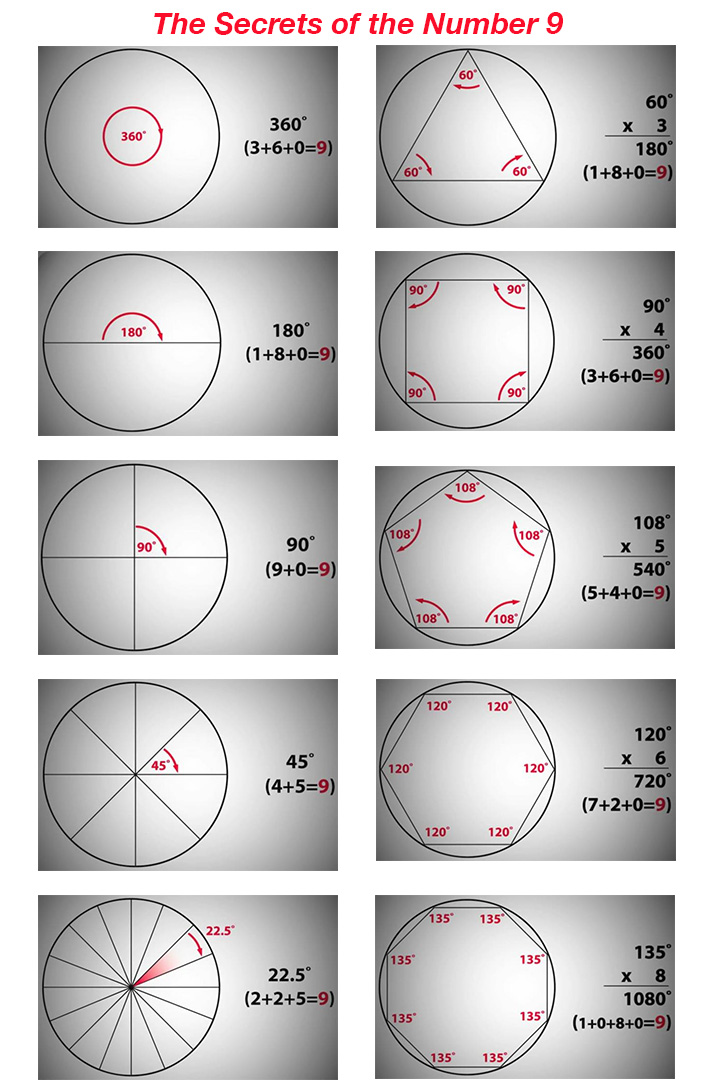Alita in Alita: Battle Angel (2019) movie said, "the woman in my memory, called me 99..."

The digital roots of 99 is 9.

Proof:
multiplication: 9*9=81, 8+1=9

In fact 9 is so powerful, anything multiple by 9, the digital roots is 9.

5*9=45, 4+5=9

332277*9=2,990,493,
2+9+9+0+4+9+3=36,
3+6=9

457683*9=4,119,147,
4+1+1+9+1+4+7=27,
2+7=9

the woman in my memory, called me 99...

Number 9 Code of Everything

Time - which is the core center of our subconscious

1440 minutes in a day = 1+4+4+0 = 9 (nine).
86,400 seconds in a day = 8+6+4+0+0=18=1+8= 9 (nine).
10,080 minutes in a week = 1+0+0+8+0= 9 (nine).
525,600 minutes in a year = 5+2+5+6+0+0 =18=1+8= 9 (nine).
minutes and seconds in a day, week, month and year will always reduce to to digital roots of nine.